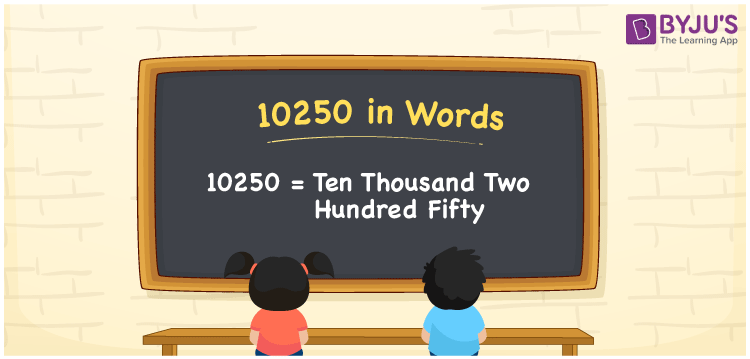# 10250 in Words

10250 in words is Ten Thousand Two Hundred Fifty. For example, Rohit purchased a camera worth Rs. 10250, then you can write the same sentence in words as “Rohit purchased a camera worth Rupees Ten Thousand Two Hundred Fifty”. The number 10250 is a cardinal number since it shows an exact quantity. Let us learn how to convert the number 10250 into words using a place value chart here.

 10250 in Words Ten Thousand Two Hundred Fifty Ten Thousand Two Hundred Fifty in numerical form 10250

## 10250 in English Words

Generally, in Mathematics, we write the numbers in words with the help of the English alphabet. Therefore, we can read the number 10250 in English as Ten Thousand Two Hundred Fifty.## How to Write 10250 in Words?

Using a place value chart, we can express the number 10250 in word form. 10250 is a five-digit number. Hence, let us make a chart of 5 columns, as shown in the below table.

 Ten Thousands Thousands Hundreds Tens Ones 1 0 2 5 0

Hence, we can write the expanded form as:

1 x Ten Thousand + 0 x Thousand + 2 x Hundred + 5 x Ten + 0 x One

= 1 x 10000 + 0 x 1000 + 2 x 100 + 5 x 10 + 0 x 1

= 10000 + 0 + 200 + 50 + 0

= 10000 + 200 + 50

= 10250

= Ten Thousand Two Hundred Fifty

Therefore, 10250 in words is written as Ten Thousand Two Hundred Fifty

Interesting way of writing 10250 in words

1 = One

10 = Ten

102 = One Hundred and Two

1025 = One Thousand Twenty-Five

10250 =Ten Thousand Two Hundred Fifty

Thus, the word form of the number 10250 is Ten Thousand Two Hundred Fifty

10250 is a natural number that precedes 10251 and succeeds 10249

• 10250 in words – Ten Thousand Two Hundred Fifty
• Is 10250 an odd number? – No
• Is 10250 an even number? – Yes
• Is 10250 a perfect square number? – No
• Is 10250 a perfect cube number? – No
• Is 10250 a prime number? – No
• Is 10250 a composite number? – Yes

## Frequently Asked Questions on 10250

Q1

### How to write 10250 in words?

10250 in words is written as Ten Thousand Two Hundred Fifty.
Q2

### Simplify 10000 + 250, and express it in words.

Simplifying 10000 + 250, we get 10250. 10250 in words is written as Ten Thousand Two Hundred Fifty.
Q3

### 10250 is a composite number. True or False.

True, 10250 is a composite number.
Test your Knowledge on 10250 in Words